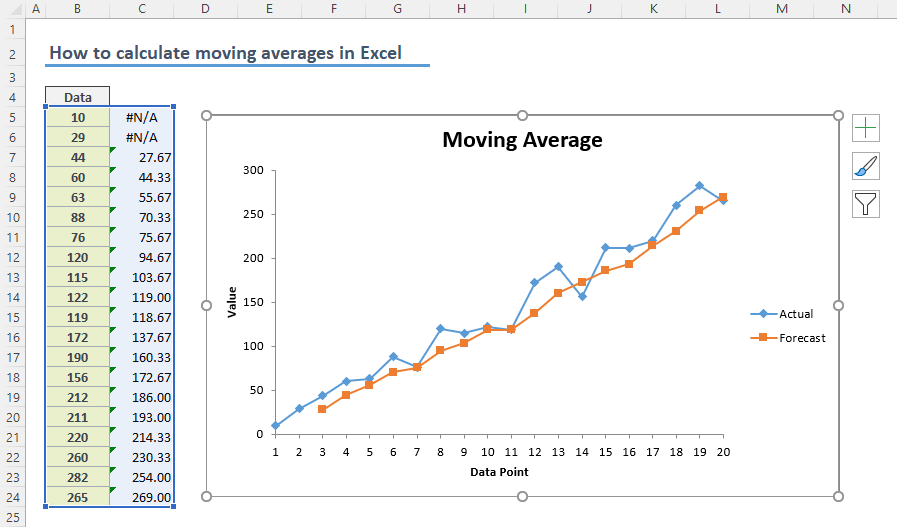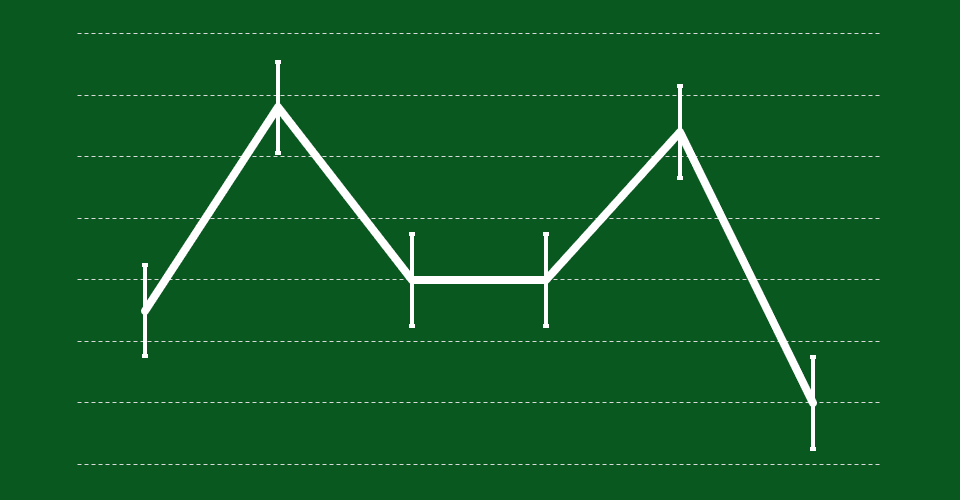## How to Perform Trend Analysis in Excel

Trend Analysis is an important tool for decision making, which allows you to analyze the historical patterns to predict future events. Excel is not shy about tools that help you in the process. In this guide, we’re going to show you How to perform trend analysis in...## How to seasonal forecast with formulas in Excel

Big or small, all business operations need forecasting. Forecasting can be either qualitative or quantitative. In the end, you should estimate future incomes and expenses to draw up a budget. Since each business sector and the company have their own dynamics, there is...## How to calculate exponential moving average in Excel

Exponential moving average option is a data smoothing approach which gives exponentially decreasing weights to values over time. In this guide, we’re going to show you how to calculate exponential moving average in Excel. Download Workbook What is exponential moving...## How to calculate weighted moving average in Excel

Weighted moving average is a data smoothing approach that gives more weight to more recent time periods. In this guide, we’re going to show you how to calculate weighted moving average in Excel. Download Workbook What is weighted moving average (WMA)? Moving average...## How to calculate simple moving average in Excel

Moving average calculations is a common way of smoothing data to reduce the effects of random, short-term fluctuations. In this guide, we’re going to show you how to calculate simple moving averages in Excel using three methods. Download Workbook What is Simple Moving...## How to calculate discrete probability in Excel

A discrete distribution describes the probability of occurrence of a random variable that can take on only a certain number of values. Common examples for this are the probabilities in a dice roll or getting a certain card in a deck of regular cards. In this guide,...## How to add error bars in Excel

Error bars are graphical representations of data variability in charts. You can add error bars into your Excel charts to provide a more comprehensive view of the data set. An error bar is usually a horizontal and/or vertical line drawn between lowest and highest error...